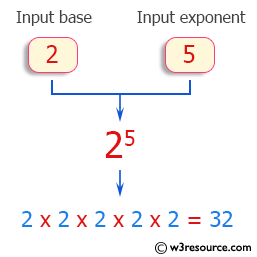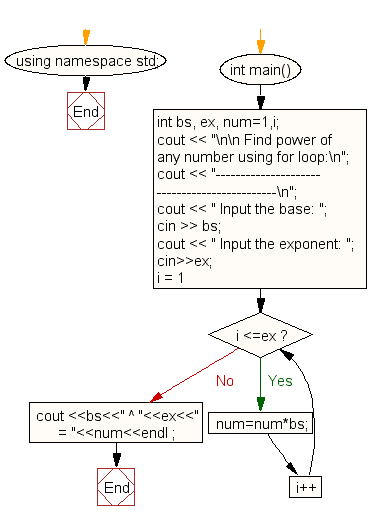﻿ C++ Exercises: Find power of any number using for loop - w3resource

# C++ Exercises: Find power of any number using for loop

## C++ For Loop: Exercise-62 with Solution

Write a program in C++ to find the power of any number using a for loop.

Pictorial Presentation:Sample Solution:-

C++ Code :

``````#include <iostream>
using namespace std;
int main()
{
int bs, ex, num=1,i;
cout << "\n\n Find power of any number using for loop:\n";
cout << "---------------------------------------------\n";
cout << " Input the base: ";
cin >> bs;
cout << " Input the exponent: ";
cin>>ex;

for (i = 1; i <=ex; i++)
{
num=num*bs;
}
cout <<bs<<" ^ "<<ex<<" = "<<num<<endl ;
}
``````

Sample Output:

``` Find power of any number using for loop:
---------------------------------------------
Input the base: 2
Input the exponent: 5
2 ^ 5 = 32
```

Flowchart:C++ Code Editor: